Question

# Selve the problem. (Spts.) 9) Multiple-choice questions on a test each have 6 possible answers, one...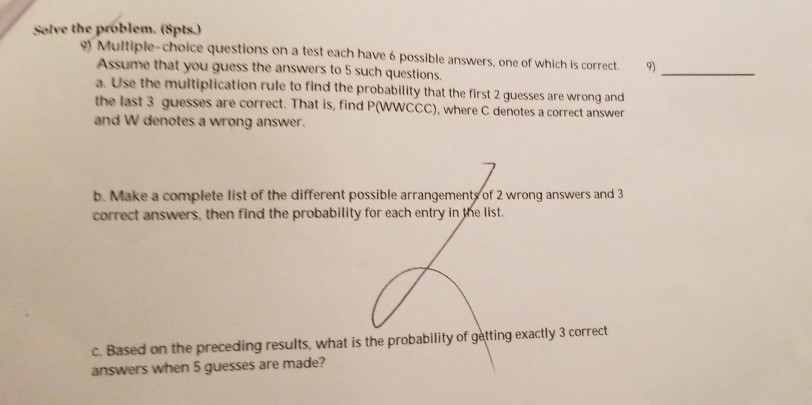Selve the problem. (Spts.) 9) Multiple-choice questions on a test each have 6 possible answers, one of which is correct. Assume that you guess the answers to 5 such questions. a. Use the multiplication rule to find the probability that the first 2 guesses are wrong and the last 3 guesses are correct and W denotes a wrong answer 9) . That is, find P(WWCCC), where C denotes a correct answer b. Make a complete list of the different possible arrangements of 2 wrong answers and 3 correct answers, then find the probability for each entry in the list. C. Based on the preceding results, what is the probability of getting exactly 3 correct answers when 5 guesses are made?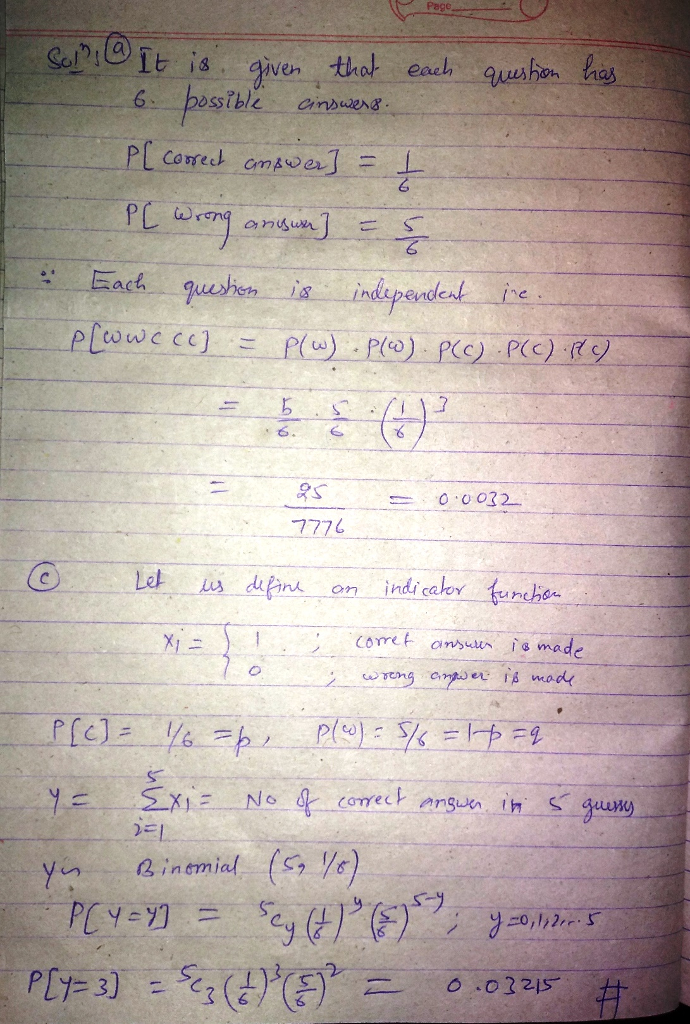#### Earn Coins

Coins can be redeemed for fabulous gifts.

Similar Homework Help Questions
• ### Multiple-choice questions each have five possible answers (a, b, c, d, e), one of which is correct

Multiple-choice questions each have five possible answers (a, b, c, d, e), one of which is correct. Assume that you guess the answers to three such questions. a. Use the multiplication rule to find P(CCW), where C denotes a correct answer and W denotes a wrong answer. P(CCW)=(Type an exact answer.) b. Beginning with CCW, make a complete list of the different possible arrangements of two correct answers and one wrong answer, then find the probability for each entry in the list. P(CCW)- see...

• ### Multiple-choice questions each have five possible answers (a, b, c, d, e), one of which is...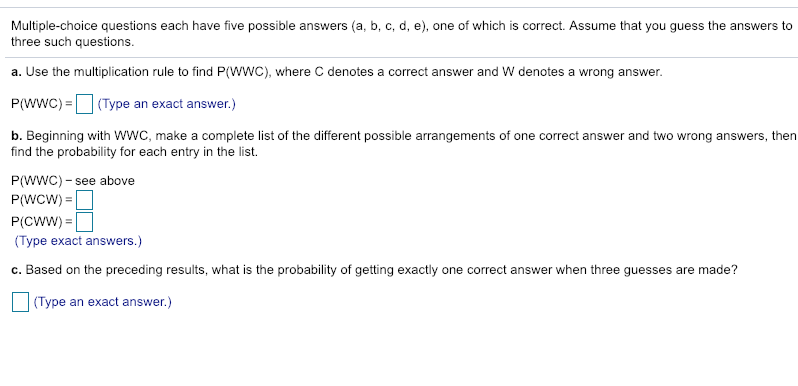Multiple-choice questions each have five possible answers (a, b, c, d, e), one of which is correct. Assume that you guess the answers to three such questions a. Use the multiplication rule to find P(WWC), where C denotes a correct answer and W denotes a wrong answer. P(WWC) (Type an exact answer.) b. Beginning with WWC, make a complete list of the different possible arrangements of one correct answer and two wrong answers, then find the probability for each entry in the...

• ### This Test: 25 pls poss Question Help Multiple-choice questions each have four possible answers (a, b,...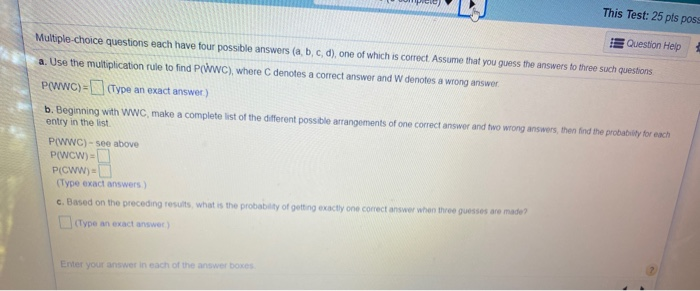This Test: 25 pls poss Question Help Multiple-choice questions each have four possible answers (a, b, c, d), one of which is correct Assume that you guess the answers to three such questions a. Use the multiplication rule to find P(WWC), where denotes a correct answer and W denotes a wrong answer, PWWC)-(Type an exact answer) b. Beginning with WWC make a complete list of the different possible arrangements of one correct answer and two wrong answers, then find the...

• ### Unsure of my answer for c. Multiple-choice questions each have four possible answers (a, b, c,...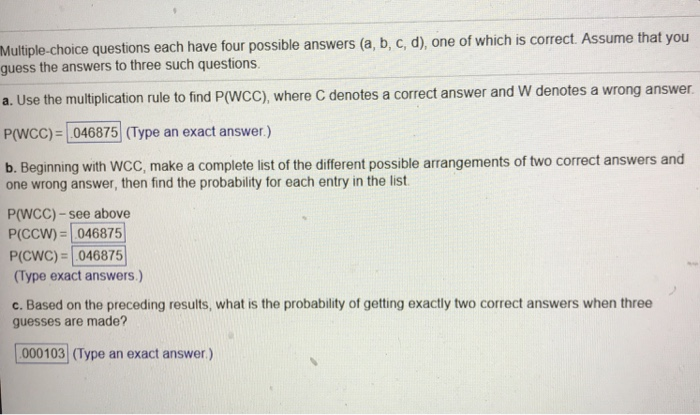Unsure of my answer for c. Multiple-choice questions each have four possible answers (a, b, c, d), one of which is correct. Assume that you guess the answers to three such questions. rect answer and W denotes a wrong answer Use the multiplication rule to find P(WCC), where C denotes a cor P/Wcc)- [046875 Type an exact answer) b. Beginning with WCC, make a complete list of the different possible arrangements of two correct answers and one wrong answer, then...

• ### ​Multiple-choice questions each have four possible answers left parenthesis a comma b comma c comma d...

​Multiple-choice questions each have four possible answers left parenthesis a comma b comma c comma d right parenthesis​, one of which is correct. Assume that you guess the answers to three such questions. a. Use the multiplication rule to find ​P(WCC​), where C denotes a correct answer and W denotes a wrong answer. P(WCC​)= ________

• ### 10. A multiple-choice test has 30 questions and each one has five possible answers, of which one...

10. A multiple-choice test has 30 questions and each one has five possible answers, of which one is correct. If all answers were guesses, find the probability ofgetting exactly four correct answers. (Points : 3).0604.1325.2552.8000

• ### A multiple choice test has 10 questions each of which has 4 possible answers, only one...

A multiple choice test has 10 questions each of which has 4 possible answers, only one of which is correct. If Judy, who forgot to study for the test, guesses on all questions, what is the probability that she will answer exactly 3 questions correctly?

• ### 10 multiple choice questions each with three possible answers only one of which is correct

10 multiple choice questions each with three possible answers only one of which is correct. A student guesses the answer to every question. (a)What is the probability that the student will answer the first six questions correctly and the rest incorrectly?(b)What is the probability that the student will answer the 1st,2nd,4th,6th,7th,and 10th questions correctly and the rest incorrectly?(c)How many ways can the student get exactly 6 correct answers?(d)What is the probability that the student answers exactly 6 questions correctly?Thank you...

• ### A multiple choice test consists of 60 questions. each question has 4 possible answers of which...

A multiple choice test consists of 60 questions. each question has 4 possible answers of which one is correct. If all answers are random guesses, estimate the probability that of 140 randomly selected seeds, exactly 100 will germinate

• ### 6. You are about to take an exam with 5 questions, four possible answers for each...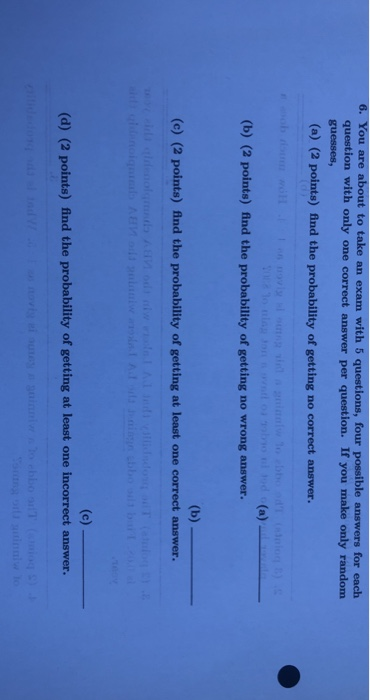6. You are about to take an exam with 5 questions, four possible answers for each question with only one correct answer per question. If you make only random guesses, (a) (2 points) find the probability of getting no correct answer. (b) (2 points) find the probability of getting no wrong answer. (c) (2 points) find the probability of getting at least one correct answer. (d) (2 points) find the probability of getting at least one incorrect answer.